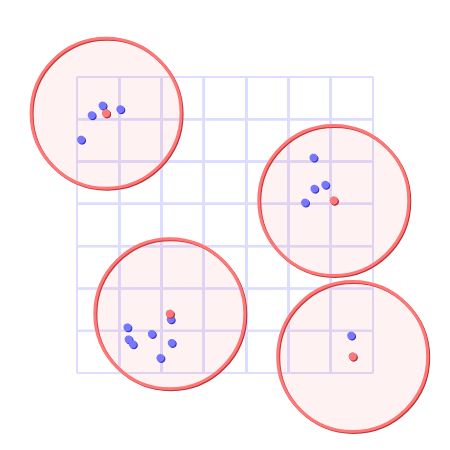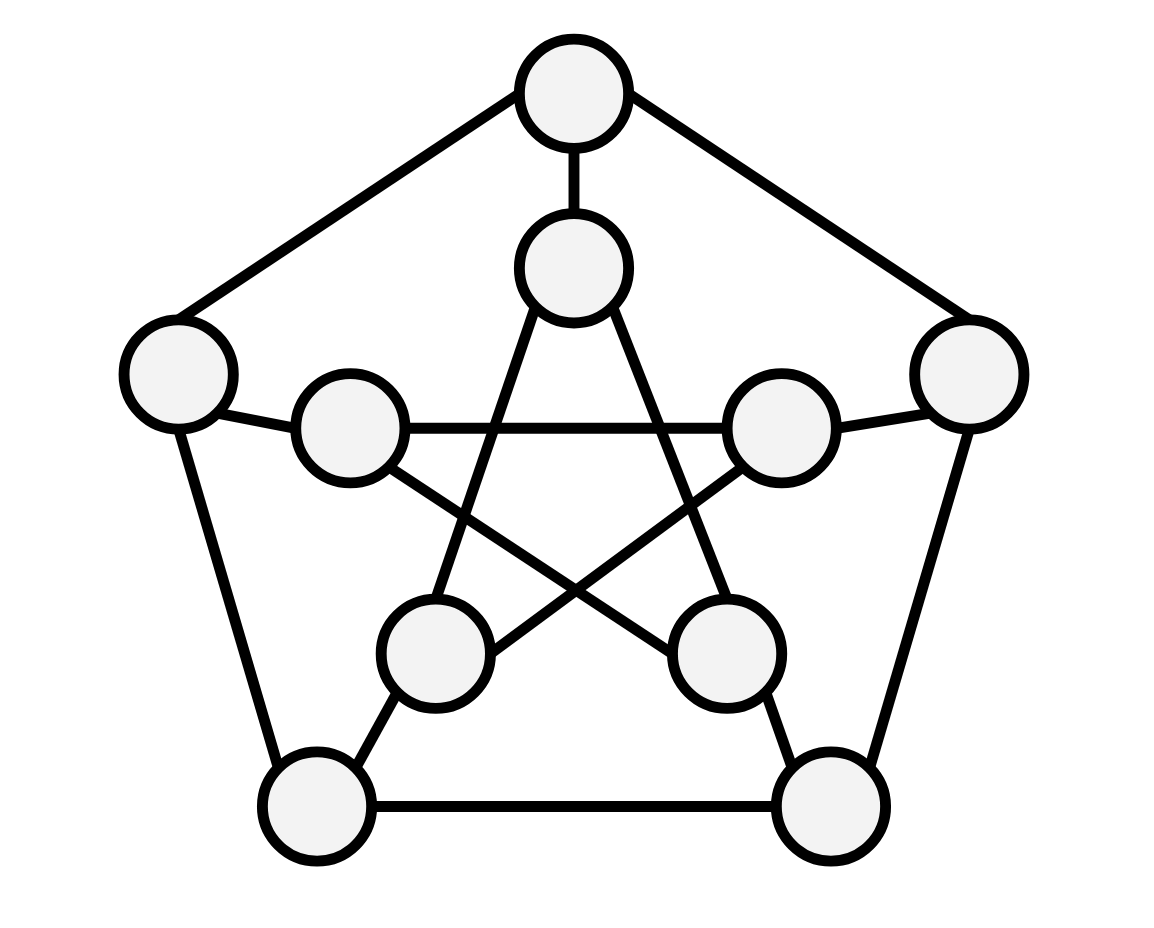# How to Share Decklists

14 Jul 2020

This is not going to be my normal kind of post, it’s not very focused, and going to be a bit rambley, as I talk about a problem I thought about one day.

Every week, Wizards of the Coast (WotC) publishes a list of decks that went “5-0” in a “league” (a player played a series of five games with that deck and won them all). Stay with me! This isn’t a post about card games. This list, however, is doctored: they want the data shown to be broad and not repetitive, so they perform some amount of deduplication on the data. This means that if two “similar” decks 5-0 a league, only one will be shown in the dump. What “similar” means is not especially clear or public, but the consensus seems to be that it’s approximately “within some number of cards different.”

This seemed to me like an interesting problem! Given a raw set of deck dumps, how could we produce such a deduplicated set of decks?

At a high level, we might envision the set of decks as a bunch of points in some space, where points are closer together if the decks are more “similar” (for some meaning of “similar” we haven’t made precise yet).With a solution being a set of decks which “cover” the set of all other decks (for some meaning of “cover” we haven’t made precise yet).To be slightly more precise, given a set $$D$$ of “decks,” we want to find a minimal set $$D^\prime \subseteq D$$ such that every $$d \in D$$ is “sufficiently close” to some $$d^\prime \in D^\prime$$.

Let’s define what we mean by “deck” and “sufficiently close.” We can represent decks as maps from the set of cards $$C$$ to some integer $$n \in \mathbb Z$$ denoting how many copies of a given card are in the deck (I know you can’t have negative numbers of cards in a deck, but it’s cleaner this way for unimportant reasons). We might also think of this as a $$\mathbb Z$$ vector indexed by $$C$$, which can be denoted $$\mathbb Z ^ C$$. That’s all to say, you should think of one of these decks as just a sequence of integers denoting how many of a given card were included, where each index corresponds to a particular card: $\langle 0, \ldots, 4 \ldots, 0 \rangle$ The sequence is finite, since there’s a finite number of Magic cards (about twenty thousand).

For “closeness,” we might say we are interested in the total number of different cards. Nobody knows for sure how WotC measures similarity, but this seems to be a reasonable way to define it. That is, the distance between two decks $$a$$ and $$b$$ is the sum of the difference of each index: $|a - b| = \sum_{c \in C} \left| a_c - b_c \right|.$ This way of measuring distance is pretty natural, and satisfies some nice properties:

1. $$|a - b| = 0$$ if and only if $$a = b$$,
2. $$|a - b| = |b - a|$$ (symmetry), and
3. $$|a - c| \le |a - b| + |b - c|$$ (triangle inequality).

These might seem kind of arbitrary things to highlight, but they’re important: a notion of distance that satisfies these properties is called a metric, and a set combined with a metric on that set is called a metric space. This particular metric we’ve stumbled on is actually well known, and is called the $$\ell_1$$-norm.

We’ll be a little abstract here, but when you hear “metric” you should just think “way of thinking about distance.” If you look at the three properties, they’re all pretty natural things you’d want from a measure of “distance.” Another way of thinking about distance is the $$\ell_2$$-norm, which you might also know as Euclidean distance: if you have two points in Euclidean space, and draw a straight line between them, the $$\ell_2$$-norm is the length of that line. In two-dimensional space, $|a - b| = \sqrt{(a_x - b_x)^2 + (a_y - b_y)^2}$ You might recognize this as the Pythagorean theorem. For our problem we’re concerned with the $$\ell_1$$-norm, as described above.

Why bother introducting this abstraction? Well, I just thought it was kind of neat, since the algorithm I ended up implementing works on any metric space. I wrote some code to solve this problem for the $$\ell_2$$-norm, which generated the pictures above, but the same code would work on this decklist problem, if you just swap out the distance function. Anyway!

We’re going to live in abstract-land for a while, but it’s sufficient to just picture this scenario and metric space (this diagram is using the $$\ell_2$$-norm).Remember that the thing we’re primarily interested in is going to be all the points “sufficiently close” to a given point, where “sufficiently close” means has distance less than some $$r$$. It turns out this thing has a name, the (closed) ball of radius $$r$$ centered at $$p$$ is the set of points $$x$$ satisfying $$|x - p| \le r$$. A “ball” is basically what you expect in most contexts, in 2D space, under the $$\ell_2$$-norm (our diagram above) a ball is a (filled in) circle. In 3D it’s a sphere. In “deck-space,” it’s the set of decks you can make from $$p$$ by adding and removing at most $$r$$ cards.

This lets us rephrase our problem a bit more generally. Given a metric space $$M$$, a finite set of points $$P \subseteq M$$ and a radius $$r$$, we want to find a set $$P^\prime \subseteq P$$ such that every $$p \in P$$ is within some ball of radius $$r$$ centered at some $$p^\prime \in P^\prime$$.

So, how do we solve this problem, in a general metric space, efficiently? We can’t! Ha-HA! Why did I bother going down this road of generalization if it was going to lead to a dead-end? Well, it’s the road I took when thinking about this problem and it’s my blog, and also it’s actually easy to show that this problem is NP-hard.

Consider a graph $$G$$:(pc: wikipedia because I didn’t want to draw my own).

The dominating set problem is this: given a graph $$G = (V, E)$$, find a set $$V^\prime$$ of vertices such that every $$v \in V$$ is adjacent to some $$v^\prime \in V^\prime$$. Hm, this already smells similar to our problem! Then define a binary function on the vertices of $$G$$: $d(a, b) = \text{the length of the shortest path between a and b}.$ It turns out $$d$$ is a metric (check this yourself, it is easy)!

If we take this metric and set $$r$$ to 1, we’ve exactly recreated the dominating set problem, which, being NP-complete, means our problem in a general metric space is also NP-complete. While this doesn’t mean that our original problem about Magic decks is NP-complete (though it’s evidence that suggests this might be the case1), it does mean that any algorithm that only uses the properties of a metric space probably doesn’t have an efficient solution.

Ok, well, how about an inefficient solution? Luckily, I recently wrote about Branch and Bound, which is a technique for solving exactly this kind of combinatorial problem.

This post would not exist without very helpful discussion from my math advisory Ilia and Forte and my Magic: the Gathering advisory Julian.

1 When asked about this, my friend Forte found a nice reduction from 3SAT to the deck problem if $$r$$ is at least four2.

2If $$r$$ is one then there actually is an efficient solution. In this case the adjacency graph is bipartite, and a dominating set of a bipartite graph can be found in polynomial time. I don’t know if there’s an efficient solution when $$r$$ is two or three.